## 4.0 Data structures with SSE

The basic SSE 32-bit floating point data type is four floating point values in what is usually considered a horizontal structure: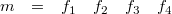It is horizontal because most SSE instructions operate on data vertically. Note that this is a 128-bit continuous block of four 32-bit floats in memory. In code this will be called vec4. For example a vertical sum between m1 and m2 can then be illustrated like this: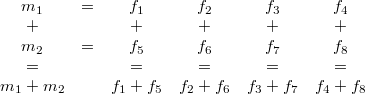How should data be then arranged into these 4xhorizontal structures? It depends on the application. Let's say the data is a set of three dimensional vectors. These could be normally arranged into array of structures (AOS) like this: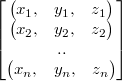or in structure of arrays (SOA) like: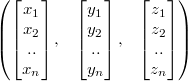For SSE these structures should be altered to incorporate the horizontal block of four floats. The first (AOS) looks then like (notice the commas):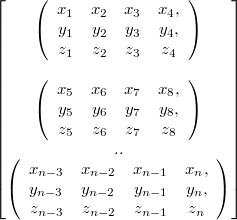Notice how for each element in structure "x" was just replaced with "x1 x2 x3 x4" and the same for y and z. Similarly the latter (SOA):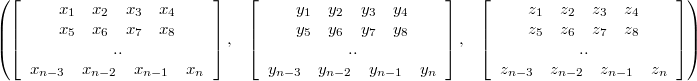Especially useful basic type is the 4x3 matrix like block in the first (AOS):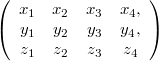Note that it is a struct with three elements: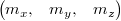It can also be obtained from SOA format just as easily by taking the nth elements from each array. The difference between the two then is that depending on the task the other uses memory cache more efficiently.

This block of four three dimensional vectors will be called mat4x3 data type in the code. To see its usefulness we will normalize these four vectors in it. To achieve that first 3 multiplications followed by 2 additions are needed vertically as follows: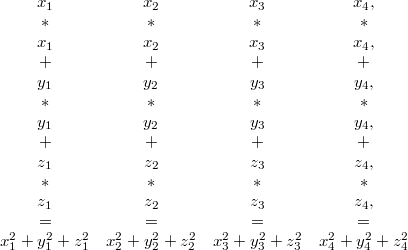Continuing from that we can execute a special SSE square root instruction to the result and multiple each three of the original components with its reciprocal to get the final result (also mat4x3, of course):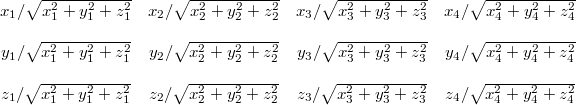This normalization can be seen in the first code example.